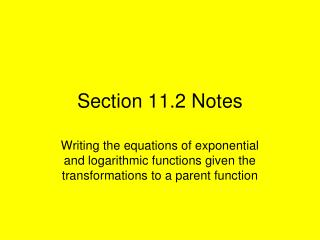DownloadDownload PresentationSection 11.2 Notes

# Section 11.2 Notes

Télécharger la présentation## Section 11.2 Notes

- - - - - - - - - - - - - - - - - - - - - - - - - - - E N D - - - - - - - - - - - - - - - - - - - - - - - - - - -
##### Presentation Transcript

1. Section 11.2 Notes Writing the equations of exponential and logarithmic functions given the transformations to a parent function

2. 11.2 Notes: Review: Write the equation of if y is reflected across the x-axis, translated left four units and down three units.

3. 11.2 Notes – Example 1 Write the equation of if y is reflected across the x-axis, translated left four units and up nine units.

4. 11.2 Notes – Example 2 Write the equation of if y is reflected across the y-axis, translated right seven units and down eight units.

5. 11.2 Notes – Example 3 Write the equation of if y is reflected across both axes and translated left eight units.

6. Practice problems: • Write the equation of reflected across the x-axis and translated left three units. • Write the equation of reflected across the y-axis, translated right one unit and up two units. 3. Write the equation of reflected across the x-axis and translated left five units.